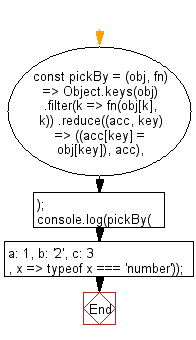# JavaScript : Create an object composed of the properties the given function returns truthy for

## JavaScript fundamental (ES6 Syntax): Exercise-97 with Solution

Write a JavaScript program to create an object composed of the properties the given function returns truthy for. The function is invoked with two arguments: (value, key).

• Use Object.keys(obj) and Array.prototype.filter()to remove the keys for which fn returns a falsy value.
• Use Array.prototype.reduce() to convert the filtered keys back to an object with the corresponding key-value pairs.
• The callback function is invoked with two arguments: (value, key).

Sample Solution:

JavaScript Code:

``````//#Source https://bit.ly/2neWfJ2
const pickBy = (obj, fn) =>
Object.keys(obj)
.filter(k => fn(obj[k], k))
.reduce((acc, key) => ((acc[key] = obj[key]), acc), {});
console.log(pickBy({ a: 1, b: '2', c: 3 }, x => typeof x === 'number'));
```
```

Sample Output:

```{"a":1,"c":3}
```

Flowchart:Live Demo:

See the Pen javascript-basic-exercise-97-1 by w3resource (@w3resource) on CodePen.

Improve this sample solution and post your code through Disqus

What is the difficulty level of this exercise?

Test your Programming skills with w3resource's quiz.

﻿

## JavaScript: Tips of the Day

Chunks an array into n smaller arrays

Example:

```const tips_chunkIntoN = (arr, n) => {
const size = Math.ceil(arr.length / n);
return Array.from({ length: n }, (v, i) =>
arr.slice(i * size, i * size + size)
);
}
console.log(tips_chunkIntoN([1, 2, 3, 4, 5, 6, 7,8], 4));
```

Output:

```[[1,2],[3,4],[5,6],[7,8]]
```